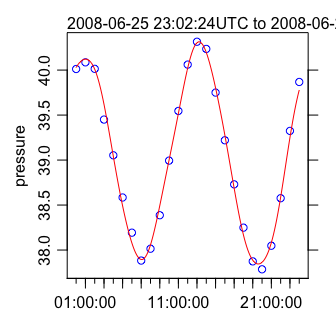# 3. Analysis of acoustic-Doppler data

#### 2021-03-27

Abstract. This vignette explains the basics of using the oce package to handle measurements made by acoustic Doppler profilers. As in the related vignette about working with data-quality flags, the material is organized to reflect typical workflow. See the main oce vignette for general information about oce. The sample code provided here relies on the oce package, loaded as follows.

library(oce)
#> Linking to GEOS 3.8.1, GDAL 3.1.4, PROJ 6.3.1

Acoustic Doppler profiling instruments are called ADCPs by some manufacturers and ADPs by others. The class name adp is used in oce, to handle both types. It is important to realize that there are many data formats for data of this type, and that the files are normally in a dense binary format that cannot be read with a text editor. It is necessary to use software to work with such files. Although oce can handle many file formats, and although its authors do their best to keep up with changes to file formats (at least for popular instruments), users will sometimes be forced to use manufacturer software to read the data, or at least convert to a format that can be manually read into R, and then converted to an adp object using the as.adp() function.

Suppose f is a character string naming a data file. In many cases,

d <- read.oce(f)

will read the file, because read.oce will look inside the file to try to guess the format. If this does not work, one ought to try e.g.

d <- read.adp(f)

which, again, will try to determine which subtype of adp file has been provided. If that fails, it might help to try a specialized function, such as read.adp.rdi() for an RDI file, read.adp.nortek() for a Nortek file, or read.adp.sontek() for a Sontek file. (Actually, each of these can handle a variety of sub-formats).

If any of these read. functions is called with just a single argument naming the file, then the entire dataset will be read in. This can be slow for large files (e.g. taking of order 0.1s per Mb), and so it can help to use the by argument, e.g. for a 138Mb file on the author’s computer,

f <- "/data/archive/sleiwex/2008/moorings/m09/adp/rdi_2615/raw/adp_rdi_2615.000"
dall  <- read.oce(f)

takes 15.2s of user time, but

d100 <- read.oce(f, by=100)

takes 0.2s. It is also possible to window the data, using the from and to arguments, which (like by) can be integers that count ensembles (or “profiles”) or a POSIX time (see ?read.adp). For example, the adp dataset provided by oce was read with

read.oce(f, from=as.POSIXct("2008-06-26", tz="UTC"),
to=as.POSIXct("2008-06-27", tz="UTC"),
by="60:00"
latitude=47.88126, longitude=-69.73433)

with f as defined above. This also illustrates that it is possibly to specify the location of an instrument; the further processing that was done to create data(adp) will be explained in due course.

The generic function summary provides a useful overview of an adp data object. For example,

data(adp)
summary(adp)

(results not shown the goal is to encourage the user to try this!) shows the sort of information normally present in an RDI file. Other manufacturer types will have somewhat different summaries, because both the metadata and the data differ from instrument to instrument. In all cases, there will be a vector holding time (named time), another holding the distance from instrument to the centre of a measurement bin, measured along a line equidistant between the beams (named distance), along with an array holding velocity (named v). (Other arrays may include, as in this RDI case, q for data quality, a for backscatter amplitude, and g for percentage goodness.)

Note that the summary reveals that instrument had been set up to record in beam coordinates, but that processing had been done to convert to enu (east-north-up) coordinates.

Exercise 1. Determine the name of the metadata item that holds the coordinate system of an adp object.

# 3 Changing coordinate systems

It is critical for analysts to be aware of the coordinate system of an adp dataset, simply because those systems are so different. In what oce calls the beam system, velocities in each of the acoustic beams are measured along the slant angle of the beam. These data may be combined (using a transformation matrix which is part of the metadata displayed with the summary() function) into what oce calls a xyz system that is Cartesian and fixed to the instrument. Since instruments record heading, pitch, and roll, the xyz velocities can be converted easily to enu velocities.

In some applications, the scientific focus is primarily on the enu data, but it is a good idea to calculate and study the data at all stages of processing. Thus, although the toEnu() function will convert data measured in any coordinate system into an enu variant, many analysts will do something along the lines of

beam <- read.oce(f)
xyx <- beamToXyz(beam)
enu <- xyzToEnu(xyz, declination=-18.1)

where the declination illustrated is the value used in creating the data(adp) dataset. (Compensating for declination is necessary because compasses are calibrated at a particular place and time, and compasses have no way of “knowing” the local angle between true north and magnetic north.)

# 4 Plotting

The generic plot() function is specialized to handle adp objects in a variety of simple but helpful ways. The type of plot is determined by the which argument. The default for which is to create a multi-panel image plot that shows time-space dependence for each velocity component. The number of panels depends on the number of beams used by the instrument, and each one has a label indicating the component name. For example,

plot(adp)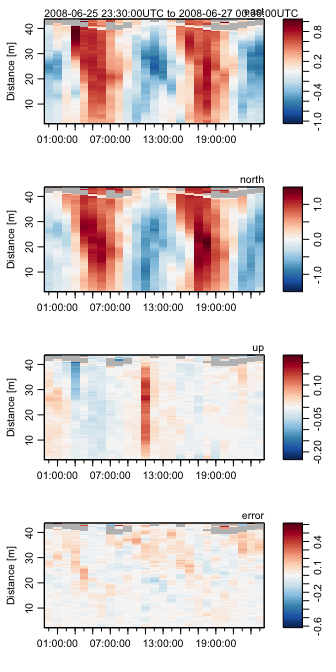has velocity panels named east, north and up, along with one named error, which is an estimate of the velocity-inference error.

Options to this plot variant can be used to control how distance from instrument is shown (which can be useful in visualizing data from upward- and downward-aligned instruments), what colours are used to represent the signals, etc.

Dozens of other plot types are also provided; see ?"plot,adp-method" for details – but be warned that a complex plot requires many arguments!

Exercise 2. Create a u-v scatterplot.

# 5 Subsetting

Adp data can be subsetted in a wide variety of ways, e.g.

plot(subset(adp, distance<20))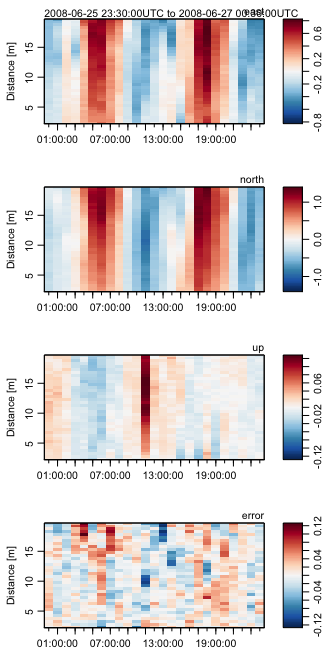plots only data within 20m of the instrument. See ?"subset,adp-method" for more on subsetting.

Exercise 3. Plot the adp data for the first half of the sampling interval.

# 6 Accessing data within adp objects

All oce objects share a common framework, with so-called “slots” for metadata, data, and processing log. It is possible to examine, and modify, the elements of each of these slots using base R constructs, but it is usually better to use oce accessor functions. The details are provided with ?"[[,adp-method" and ?"[[<-,adp-method", but a few examples should suffice to sketch the general pattern.

For example,

time <- adp[["time"]]
distance <- adp[["distance"]]

extracts the times and distances of the profiles within data(adp), while the array holding the velocities is extract with

v <- adp[["v"]]

Altering values uses the [[ to the left of the assignment operator, but this is not commonly required in simple work, so it is best for readers to focus first on accessing data. The exercises may help with this.

Some of the fields contained in adp objects, particularly the amplitude, correlation, and percent good fields (a, q, and g) are stored as raw-type. This is done primarly to save memory space, as expanded all of those fields into numeric types can be slow and they get large quickly. However, to actually work with the numbers, users can extract a “numeric” version by supplying the second argument to the [[ function, e.g.

a <- adp[["a", "numeric"]]

This has the advantage of returning an object with the same matrix dimensions as the original (note that functions like as.numeric() typically discard dimension information, thereby converting an array to a vector).

Exercise 4. Plot a time series of mid-depth and depth-averaged eastward velocities.

Exercise 5. Perform an eigen analysis of eastward and northward velocity.

Exercise 6. Plot a time series of pressure variations, to reveal tidal height.

# 7 Solutions to exercises

Exercise 1. Determine the name of the metadata item that holds the coordinate system of an adp object.

Solution.

sort(names(adp[["metadata"]]))

(results not shown), after which a keen eye will notice that two elements relate to coordinates:

adp[["originalCoordinate"]]
#>  "beam"
#>  "enu"

and comparison with the output of summary(adp) reveals that the former is the coordinate system in which the data were originally measured, while the latter is the coordinate system of the transformed data. Using

processingLogShow(adp)
#> * Processing Log
#>
#>     - 2019-08-12 15:29:36 UTC: read.oce("/data/archive/sleiwex/2008/moorings/m09/adp/rdi_2615/raw/adp_rdi_2615.000", ...)
#>     - 2019-08-12 15:29:36 UTC: beamToXyzAdp(x = beam)
#>     - 2019-08-12 15:29:36 UTC: xyzToEnuAdp(x, declination=-18.1, debug=0)

reveals the steps in the data transformation.

Exercise 2. Create a u-v scatterplot.

Solution.

plot(adp, which="uv")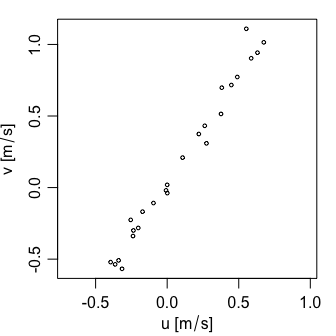Exercise 3. Plot the adp data for the first half of the sampling interval.

Solution.

plot(subset(adp, time < median(adp[["time"]])))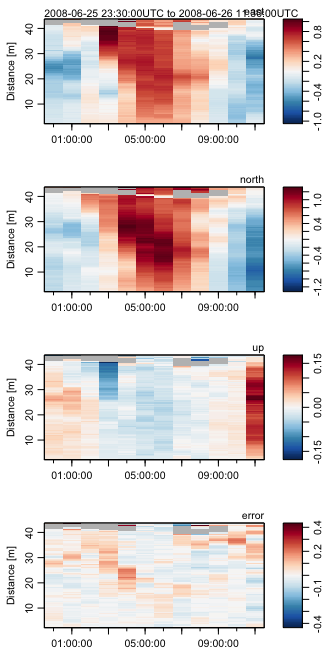Exercise 4. Plot a time series of mid-depth and depth-averaged eastward velocities.

Solution.

time <- adp[["time"]]
# The second index is for bin number, the third for beam number
midIndex <- dim(v)/2
eastMid <- v[, midIndex, 1] # third index is beam
oce.plot.ts(time, eastMid, ylab="Eastward velocity [m/s]")
## Depth mean; note that na.rm, is passed by apply() to mean()
eastMean <- apply(v[,,1], 1, mean, na.rm=TRUE)
lines(time, eastMean, col=2)
legend("top", lwd=1, col=1:2,
legend=c(paste("At", distance, "m"), "Depth Avg"))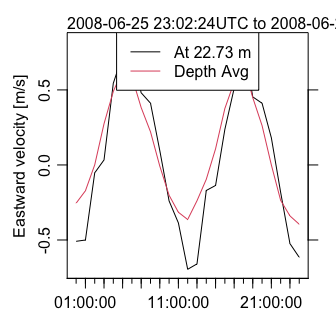Here, oce.plot.ts() is used instead of the basic R function plot(), because it provides a margin note on the detailed time interval.

Exercise 5. Perform an eigen analysis of eastward and northward velocity.

Solution.

u <- adp[["v"]][,,1]
ok <- is.finite(u) & is.finite(v) # remove NA values
u <- u[ok]
v <- v[ok]
eigen(cov(data.frame(u, v)))
#> eigen() decomposition
#> $values #>  0.48350161 0.01557049 #> #>$vectors
#>           [,1]       [,2]
#> [1,] 0.5441538 -0.8389855
#> [2,] 0.8389855  0.5441538

Note that this action is equivalent to principal component analysis, and it might be wiser to use

pr <- prcomp(data.frame(u,v))

to do the analysis, which yields the same principal axes

pr
#> Standard deviations (1, .., p=2):
#>  0.6953428 0.1247818
#>
#> Rotation (n x k) = (2 x 2):
#>         PC1        PC2
#> u 0.5441538 -0.8389855
#> v 0.8389855  0.5441538

but has the advantage of having associated methods, including for plotting.

Exercise 6. Plot a time series of pressure variations, to reveal tidal height.

Solution.

time <- adp[["time"]]
oce.plot.ts(time, pressure)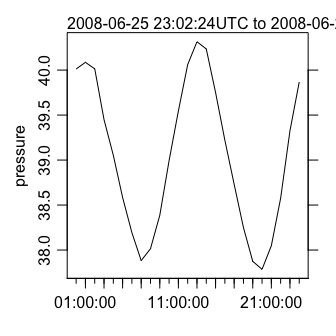As a matter of interest, a tidal analysis may be done with

m <- tidem(as.sealevel(pressure, time))
#> Note: the tidal record is too short to fit for constituents:  SA SSA MSM MM MSF MF ALP1 2Q1 SIG1 Q1 RHO1 O1 TAU1 BET1 NO1 CHI1 PI1 P1 S1 PSI1 PHI1 THE1 J1 SO1 OO1 UPS1 OQ2 EPS2 2N2 MU2 N2 NU2 GAM2 H1 H2 MKS2 LDA2 L2 T2 S2 R2 K2 MSN2 ETA2 MO3 M3 SO3 MK3 SK3 MN4 M4 SN4 MS4 MK4 S4 SK4 2SK5 2MN6 M6 2MS6 2MK6 2SM6 MSK6 M8

where the list of undetermined tidal constituents is long, because this tidal record is so short (see ?tidem). The fitted constituents are as follows

summary(m)
#> tidem summary
#> -------------
#>
#> Call:
#> tidem(t = as.sealevel(pressure, time))
#> RMS misfit to data:  0.06615227
#>
#> Fitted Model:
#>         Freq Amplitude Phase       p
#> Z0    0.0000   39.0465   0.0 < 2e-16 ***
#> K1    0.0418    0.0689 334.9   0.089 .
#> M2    0.0805    1.1730 203.8 4.6e-16 ***
#> 2MK5  0.2028    0.0312 112.8   0.417
#> 3MK7  0.2833    0.0265  87.8   0.618
#> ---
#> Signif. codes:  0 '***' 0.001 '**' 0.01 '*' 0.05 '.' 0.1 ' ' 1
#> * Processing Log
#>
#>     - 2021-03-27 22:29:13 UTC: create 'tidem' object
#>     - 2021-03-27 22:29:13 UTC: tidem(t = as.sealevel(pressure, time))

(Note that it fitted for M2, but not S2, because the Rayleigh criterion prevents inferring both, and the conventional tidal analysis favours M2 over S2 if the time series is too short to fit for more than one semi-diurnal constituent.)

A useful step might be to draw a tidal fit along with the data. It makes sense to construct a finer time grid, to get a smooth curve, as in the following (see ?predict.tidem).

oce.plot.ts(time, pressure, type="p", col="blue")
timePredict <- seq(min(time), max(time), length.out=200)
pressurePredict <- predict(m, timePredict)
lines(timePredict, pressurePredict, col="red")`